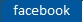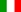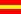Good navigation with NihilScio!NS NihilScio
Home  Educational search engine Auxilium
 Full conjugation of á     é     è     ì     í     ò ó     ù     ú     ü     ñ     çItEs
Indice delle schede di Inglese
 Words found: INFINITIVE Present to assume 1) I assume = assume: Verbo attivo INDICATIVE Present 1 pers. sing. (Details below)
 - Full conjugation of assume
 Present I assume You assume He/She/It assumes We assume You assume They assume
 Present let me assume assume let him assume let us assume assume let them assume
Conjugation of: assume , assumed , assumed
transitivo - attiva